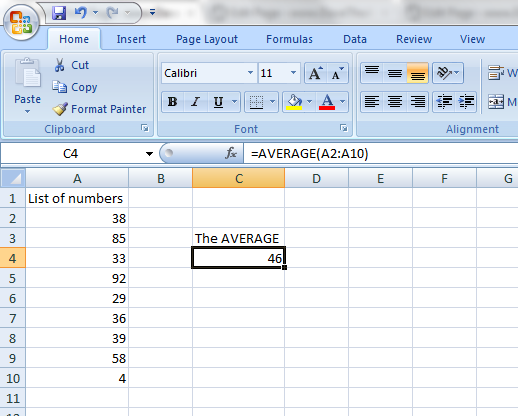## Average Calculator: calculate average of a sample or population

### How to calculate average in excel (or other spreadsheet)

In a spreadsheet like excel, we can use the build-in mathematical function AVERAGE().
The AVERAGE function can be used with a series of parameters like

=AVERAGE( number_1, number_2, ... number_255 )### Calculate average of a range (excel)

The AVERAGE function can be used with a range of cells as parameter like

= AVERAGE(A2 : A10) , which will calculate the average of all values from cell A2 to cell A10.### Calculate Standard deviation in excel

Use the build-in statistical function STDEV() to calculate the standard deviation of a set of data in excel. The STDEV() function can be used with a set of values or with a range of cells as parameter.

example:
=STDEV( number_1, number_2, ... number_255 )
=STDEV(A1 : A99)